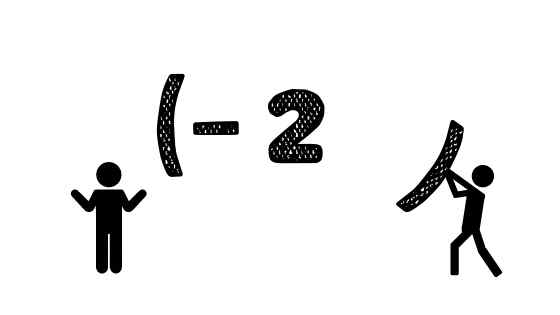# Brackets in Math: They Carry a Lot of WeightEvery symbol, every line, every semicolon matters when it comes to math. One dot in the wrong spot can change the meaning of the mathematical sentence and consequently the whole solution to the problem.

How many times students loose points on their math evaluations simply because they miss a negative sign or square a number wrong, or because of not following the order of operations correctly or… forgetting about the brackets where they are necessary. However, brackets in math are important and cannot be overlooked.

Brackets. Let’s discuss their importance in math and how to help students remember the most common cases where brackets are absolutely crucial.

#### The order of operations

Students start to really understand the importance of brackets in math when they start learning the order of operations. Now, where does the order of operations come from and who invented it? Many modern mathematicians agree that it was developed at the same time the algebraic notation was discovered and introduced, which was in the 1600s. However, even during symbolic algebra times it was clear to most that multiplication had to precede addition in order to arrive at the correct solution.

Today we learn and eventually use the order of operations rather naturally. In the 20th century an acronym to represent the order of operations was created – BEDMAS: Brackets, Exponents, Division, Multiplication, Addition, Subtraction.

As we can see, whenever an algebraic expression contains brackets – the operation in brackets should be done prior to any other operations. That is, of course, if the operation in brackets is possible. For example, in the expression 2 + (2x – 1) even though there are brackets and there is an operation within them, we cannot really perform it, since the terms that are being added are not like terms as of them contains a variable and the other one is just a constant. Thus, in this expression, we can “drop” the brackets and then simplify:

2x + 2 – 1 = 2x + 1.

It is important to practice and get really good at BEDMAS as it serves as a foundation for many-many other concepts, both simple and complex.

#### Brackets and negative numbers

Brackets are extremely important when performing operations on negative numbers.

One of the most common mistakes students make is forgetting to put a negative number in brackets when raising it to an even number exponent.

Here is why it is so important:

(-2)5 means that (-2) has been multiplied by itself 5 times

(-2) × (-2) × (-2) × (-2) × (-2) which equals +32

whereas

-25 means -(25), therefore only 2 (without the negative) has been multiplied by itself 5 times and the result is then multiplied by (-1)

– (2 × 2 × 2 × 2 × 2) which equals -31

Another situation when it is important to put a negative number in brackets is when there is a double negative sign. For example, an expressions such as 242-(-12) means we are subtracting a negative value of 12 (which means adding 12) from the product of 24 and 2.

After evaluating this expression, the answer is 48 + 12 = 60.

The situation described above is very common when multiplying, dividing, adding and subtracting positive and negative integers, fractions and decimals. It is also applied to situations where a negative sign is being distributed over an expression in brackets, like this: (3x+1) = -3x – 1

#### Distributive property of multiplication

Brackets bear yet another important meaning once students start working with algebraic expressions and equations involving variables.

A farmer is building a fence around the rectangular field. The length of the fence is 100 m longer than the width. The total area of the field is 600 square meters. What are the actual dimensions of the field and how much fencing is needed in total?

We do not know how wide or how long the field is, but we can use a variable to represent the width and then the expression representing the length will be 100 more meters (addition).

Let x be the width, then the length is (x+10) – note how the whole expression represents the length, this is why we put it in brackets, to show that this is all one dimension.

Now, if we apply the Area of a rectangle is A = lw

So, using the information at hand, our equation will look like this:

600 = x(x+100)

Notice how brackets make a big difference in how this equation is set up and how we will eventually arrive at the solution. If set up correctly as above, the variable x needs to be distributed over every single term inside the bracket.

If it had been set up without the brackets, 100 would remain as a constant.

Just like in programming, every symbol counts and one extra or missing bracket can make everything go wrong so that the code does not work, the same goes with math in general. Correct notation, form and appropriate symbols are extremely important in order to obtain the desired outcome. This is why brackets in math require special attention. Teaching kids how to organize math concepts and why it is important will help them be more successful and confident in math for years to come.

0